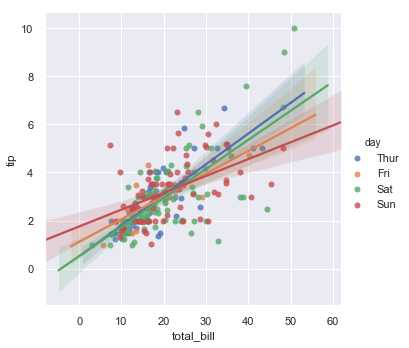# 轻松用 Seaborn 进行数据可视化

Seaborn recommend

Seaborn 是一个数据可视化库，可帮助在Python中创建有趣的数据可视化。 大多数数据分析需要识别趋势和建立模型。 本文将帮助您开始使用 Seaborn库创建数据可视化。

Seaborn 是一个出色的数据可视化库，它让我们的生活变得轻松。 首先，您应该在 jupyter notebook 中键入以下命令。

import pandas as pd # Pandas
import numpy as np # Numpy
import matplotlib.pyplot as plt # Matplotlibrary
import seaborn as sns # Seaborn Library
%matplotlib inline
sns.set()


## 直方图 （Distplot）

sns.distplot（）结合直方图并绘制核密度估计图。 这里 bin 区间大小是自动计算的。

$sns.distplot(data[“variablename”])$

# Load the Dataset in Python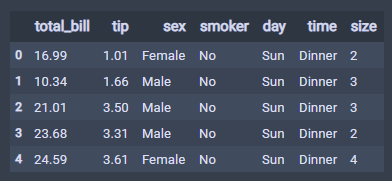sns.distplot(tips["total_bill"], bins=16, color="purple")
# Binsize is calculated using square-root of row count.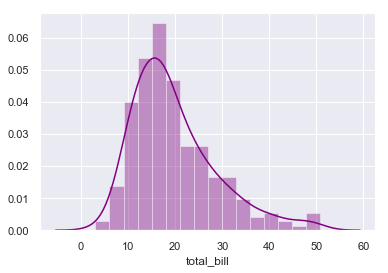• sns.distplot — 这个命令将启动 distplot 的初始创建

• tips[“total_bill”] — 从 tips 数据集（数据框）中取出列（total_bill）。 在这里，我们应该观察一下，可以使用方括号来拉取列值，并且列名应该用引号括起来（双引号/单引号）都被接受。

## 联合分布图 （Jointplot）

$sns.jointplot(x = , y =, data=)$

sns.jointplot(x = "total_bill", y = "tip", data = tips, color="purple")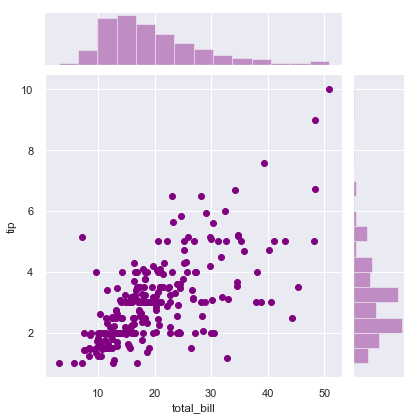### Jointplot :: kind =”hex”

$sns.jointplot(x = , y =, data=, kind=”hex”)$
# Jointplot - Scatterplot and Histogram
sns.jointplot(x = "total_bill", y = "tip", data = tips, kind ="hex",
color="lightcoral")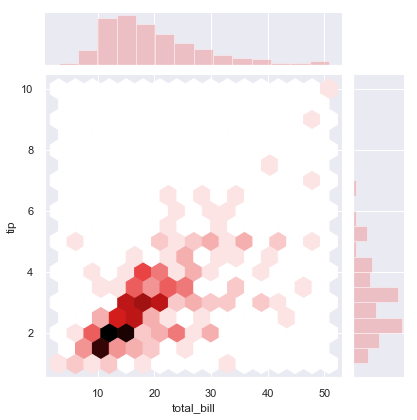kind 参数值可以是以下取值：

 kind : { “scatter” “reg” “resid” “kde” “hex” }

### Jointplot :: kind =”kde”

# Jointplot - Scatterplot and Histogram
sns.jointplot(x = tips["total_bill"], y = tips["tip"],kind = "kde",
color="purple") # contour plot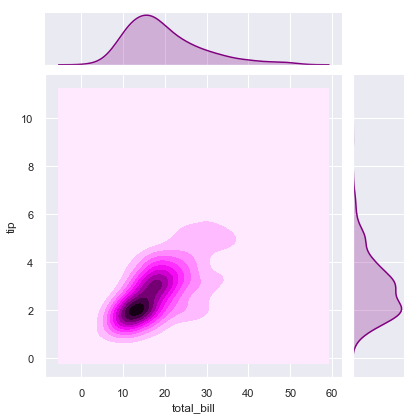## 矩阵图 （Pairplot）

$sns.pairplot(“dataframe”)$
# Pairplot of Tips
sns.pairplot(tips, hue = "sex", palette="Set2")
# this  will color the plot gender wise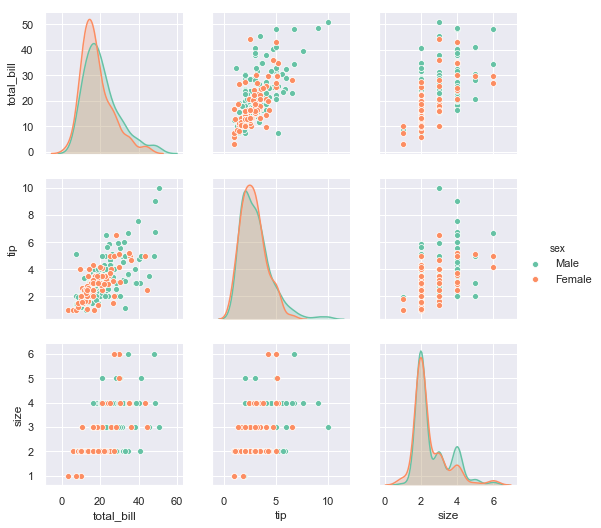hue = “sex” — 设置为按不同的性别进行着色

palette = “Set2” - “Set2” 是颜色的一个系列。

## 条形图 （Barplot）

$sns.barplot(x = , y =, data=)$
# Barplot
sns.barplot(x ="sex" , y ="total_bill" , data=tips)
# Inference - Total Bill Amount for males is more than Females.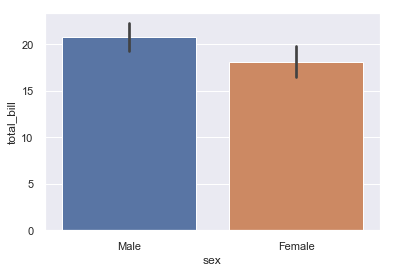# Lets Plot Smoker Vs Total Bill :: The purpose is to find out if
# Smokers pay more bill than Non Smokers
sns.barplot(x = "smoker", y = "total_bill", data =tips)
# Inference - More Bill for Smokers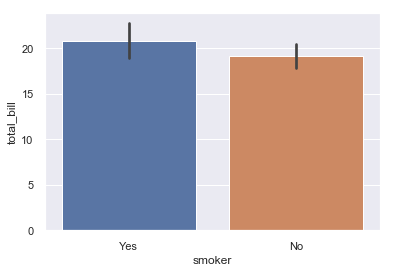# Lets Find If There is more Bill In Weekend or Weekdays
sns.barplot(x = "day", y = "total_bill", data =tips)
# People tend to visit more on weekends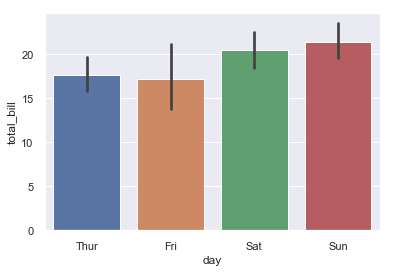## 箱形图 （Boxplot）

• Minimum 最小值
• First Quartile 1/4 值
• Median (Second Quartile) 中位数
• Third Quartile 3/4 值
• Maximum 最大值

$sns.boxplot(x = , y =, data=)$
# Boxplot
sns.boxplot(x = "day", y = "total_bill", data=tips)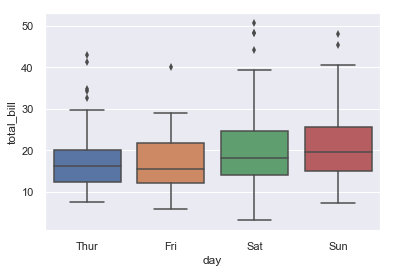# Add hue to split the barplot. Making it more fancier
sns.boxplot(x = "day", y = "total_bill", data=tips, hue = "smoker")
# On Friday people have more bill if they are a Non smoker vs smoker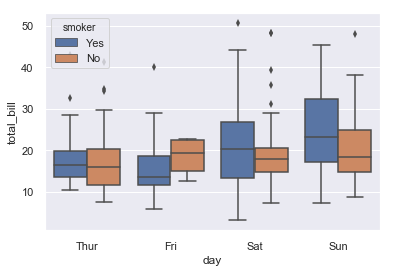hue =“smoker”： - 它为吸烟者和非吸烟者创造了一个箱线图。 例如： 在星期五的情况下，可以清楚地看到，与当天的吸烟者相比，非吸烟者的食物费用更多。

# Violin Plots
sns.violinplot(x = "day", y = "total_bill", data = tips)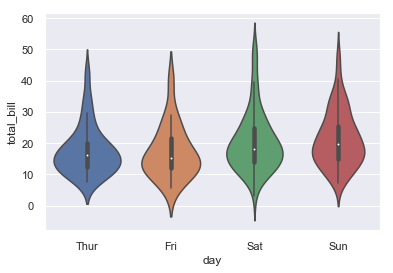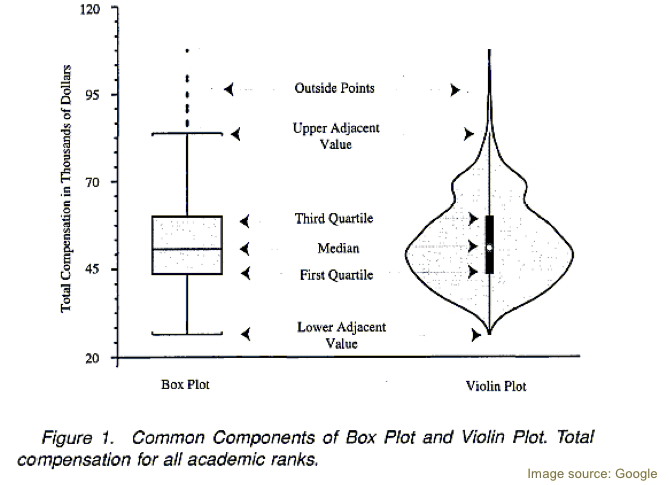## LM Plot

sns.lmplot 是绘制一个在散点图中进行线性回归拟合的直线。 它遵循普通的最小二乘法，线代表最佳拟合线。 为了更好地理解这一点，建议进一步了解一下线性回归的相关知识。

# LM PLot
sns.lmplot(x = "total_bill", y = "tip", data = tips, hue="day")